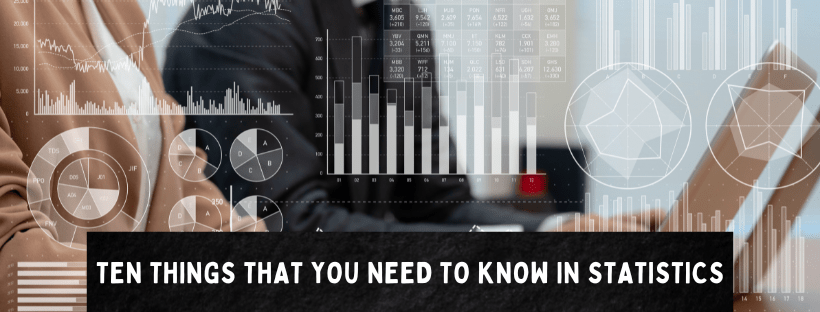## Ten Things That You Need To Know In Statistics: The Fundamentals of Statistics

Many people find statistics confusing enough mainly due to the abundance of assumptions, theorems, terms, etc. However, if you’re planning to enter the field of data science, it’s a must to have a solid understanding of the fundamentals of statistics. It’s important...## Being Good At Math To Be A Good Data Scientist?

Being good at math is an important skill for a data scientist to have, as data science involves the use of mathematical and statistical concepts and techniques to analyze and interpret data. However, the level of math proficiency required can vary depending on the...